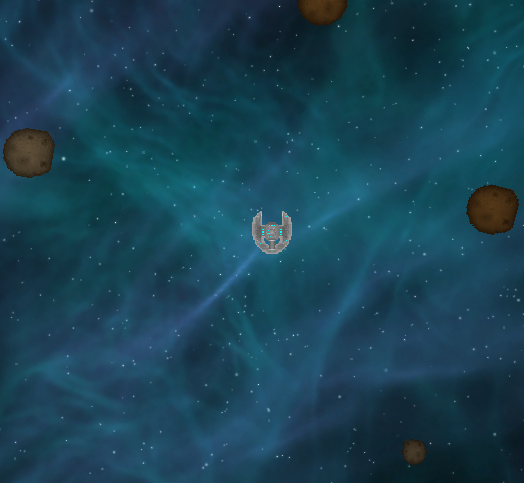# [2d] Rotating to target position.

Hi,
I have been trying to do this for a good few hours now so i decided to try get some help. My game is 2d and the camera is at (0,0,-5) im trying to get an entity to look at me and follow me where ever i go but when i try to do it with the following code it flips the sprite and rotates him on a different axis and moves him off the playing plane (or other complications).

here is some stuff i have tried and a picture of how the game works.

``````			float angle = Mathf.Atan2(destination.x, destination.y) * Mathf.Rad2Deg;
transform.rotation = Quaternion.AngleAxis(angle, Vector3.forward);//this just made him go across the x axis.

Vector2.MoveTowards(transform.position,selectedAsteroid.transform.position,1 * Time.deltaTime);
//this just makes him go forward in the direction he is pointing.

neededRotation = Quaternion.LookRotation (transform.position - destination, Vector3.forward);
transform.rotation = Quaternion.Slerp (transform.rotation, neededRotation, rotationSpeed * Time.deltaTime);
//this one makes him go off on to the wrong axis and rotate around me on the z axis.
````````````			neededRotation = Quaternion.LookRotation(Vector3.forward, destination - transform.position);